Showing first {{hits.length}} results of {{hits_total}} for {{searchQueryText}}{{hits.length}} results for {{searchQueryText}}

Keine Suchergebnisse

Minted is a package that allows formatting and highlighting source code in LaTeX. This article explains how to use it. Contents 1 Introduction 2 Basic usage 3 Including code from a file 4 One-line code 5 Custom lexers 6 Colours and style sheets 7 Captions, labels and the list of listings 8 Reference guide 9 Further reading  Introduction Using the package minted is straightforward. \documentclass{article} \usepackage[utf8]{inputenc} \usepackage[english]{babel}   \usepackage{minted}   \begin{document} \begin{minted}{python} import numpy as np   def incmatrix(genl1,genl2): m = len(genl1) n = len(genl2) M = None #to become the incidence matrix VT = np.zeros((n*m,1), int) #dummy variable   #compute the bitwise xor matrix M1 = bitxormatrix(genl1) M2 = np.triu(bitxormatrix(genl2),1)   for i in range(m-1): for j in range(i+1, m): [r,c] = np.where(M2 == M1[i,j]) for k in range(len(r)): VT[(i)*n + r[k]] = 1; VT[(i)*n + c[k]] = 1; VT[(j)*n + r[k]] = 1; VT[(j)*n + c[k]] = 1;   if M is None: M = np.copy(VT) else: M = np.concatenate((M, VT), 1)   VT = np.zeros((n*m,1), int)   return M \end{minted} \end{document}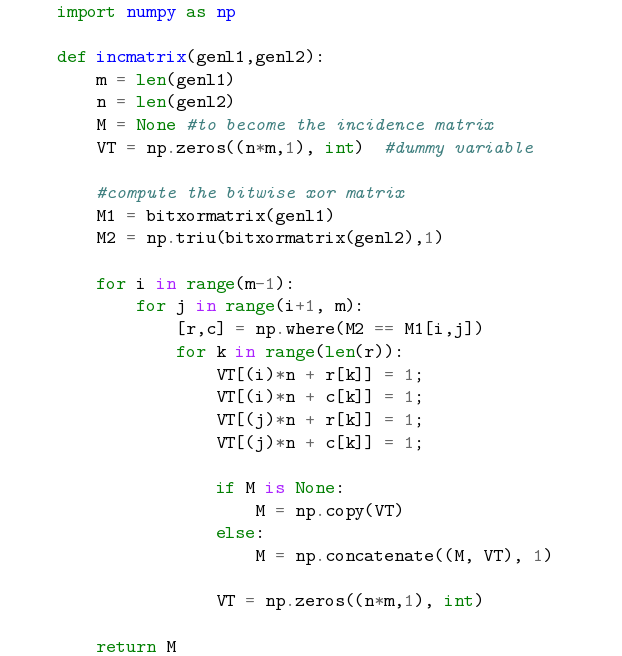There are two important commands here. In the preamble the package is imported by \usepackage{minted} then the tags \begin{minted}{python} and \end{minted} delimit an environment that print the text verbatim in monospaced fonts and also colour comments, keywords and functions. The parameter python is the programming language the source code is written in. minted supports over 150 programming and markup languages as well as configuration files, see the reference guide for a list of supported languages. Note: For minted to work with you local LaTeX distribution an additional program called Pygments must be installed, Overleaf can save you the trouble of installing it and having to run special commands to compile your document. Documents that use minted work "out of the box" in Overelaf.   Open an example of the minted package in Overeaf  Basic usage There are some options in the minted environment that change the visual aspect of the code. \begin{minted} [ frame=lines, framesep=2mm, baselinestretch=1.2, bgcolor=LightGray, fontsize=\footnotesize, linenos ] {python} import numpy as np   def incmatrix(genl1,genl2): m = len(genl1) n = len(genl2) M = None #to become the incidence matrix VT = np.zeros((n*m,1), int) #dummy variable   #compute the bitwise xor matrix M1 = bitxormatrix(genl1) M2 = np.triu(bitxormatrix(genl2),1)   for i in range(m-1): for j in range(i+1, m): [r,c] = np.where(M2 == M1[i,j]) for k in range(len(r)): VT[(i)*n + r[k]] = 1; VT[(i)*n + c[k]] = 1; VT[(j)*n + r[k]] = 1; VT[(j)*n + c[k]] = 1;   if M is None: M = np.copy(VT) else: M = np.concatenate((M, VT), 1)   VT = np.zeros((n*m,1), int)   return M \end{minted}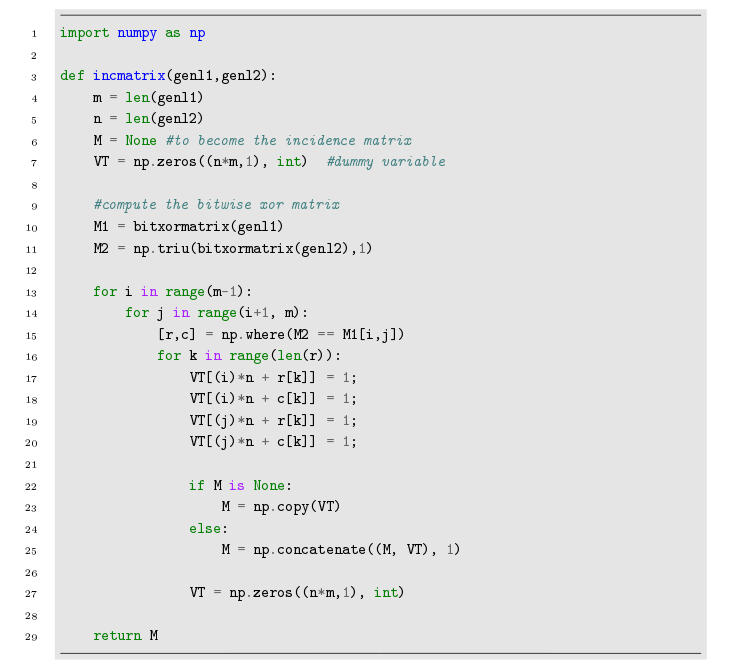This is the example presented in the introduction, but the opening delimiter for the environment now has the syntax \begin{minted}[...]{python}. Inside the brackets several comma-separated parameters in the form key=value are set: frame=lines Draws two lines, one on top and one at the bottom of the code to frame it. Other possible values are leftline, topline, bottomline and single. framesep=2mm The frame separation is set to 2mm. Other length units can be used. baselinestretch=1.2 Interlining of the code set to 1.2. bgcolor=LightGray Background colour set to light grey. You need to import xcolor for this to work. See Using colours in LaTeX to learn more about colour manipulation. fontsize=\footnotesize Font size set to footnotesize. Any other font size can be set. linenos Enables line numbers. Other options that may be useful are: mathescape. Enables math mode in code comments. rulecolor. Changes the colour of the frame. showspaces. Enables a special character to make spaces visible.   Open an example of the minted package in Overleaf  Including code from a file Code is usually stored in a source file, therefore a command that automatically pulls code from a file becomes very handy. \documentclass{article} \usepackage[utf8]{inputenc} \usepackage[english]{babel}   \usepackage{minted}   \begin{document} The next code will be directly imported from a file:   \inputminted{octave}{BitXorMatrix.m} \end{document}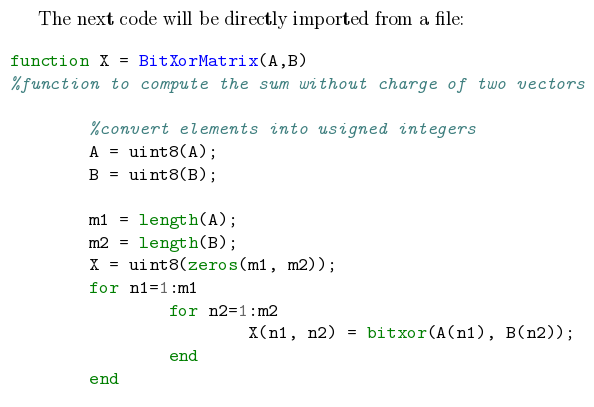The command \inputminted{octave}{BitXorMatrix.m} imports the code from the file BitXorMatrix.m, the parameter octave tells LaTeX the programming language of the code. This command can take two extra parameters to import only part of the file; for instance, to import code from the line 2 to the line 12, the command becomes: \inputminted[firstline=2, lastline=12]{octave}{BitXorMatrix.m}   Open an example of the minted package in Overleaf  One-line code If you need to input only a line of code, the command \mint, whose syntax is presented in the next example, will do the trick. One-line code formatting also works with minted. For instance, a simple html sample like this: \mint{html}|

## Something here

| can be properly formatted.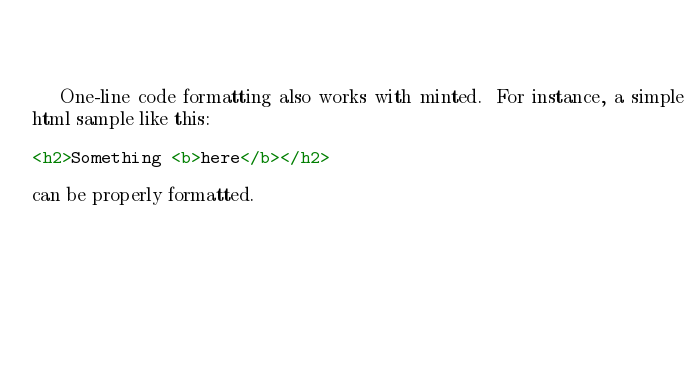The parameter in between braces set the programming language (markup language in this case), the actual text to be formatted is delimited by the character |.   Open an example of the minted package in Overleaf  Custom lexers By default, minted supports only languages with lexers that are already installed or registered with pygmentize. If you have written a custom lexer, or want to use a lexer for a language that's not yet been installed on Overleaf, you can still use it in your own Overleaf project using the approach mentioned here. Say for example you have defined a lexer in the file nl-lexer.py, containing the class NetLogoLexer for the NetLogo language. Upload nl-lexer.py to your Overleaf project, and then specify nl-lexer.py:NetLogoLexer as the "language name" when using minted. For example: \begin{minted}{nl-lexer.py:NetLogoLexer -x} ... your code here ... \end{minted} Here's another example for the ImageJ Macro language.  Colours and style sheets The colour schemes for code highlighting are saved in style sheets. You can create your own or use one already available in your LaTeX distribution. See the reference guide for a list of stylesheets included in Overleaf. \documentclass{article} \usepackage[utf8]{inputenc} \usepackage[english]{babel}   \usepackage{minted} \usemintedstyle{borland}   \begin{document} \begin{minted}{python} import numpy as np   def incmatrix(genl1,genl2): m = len(genl1) n = len(genl2) M = None #to become the incidence matrix VT = np.zeros((n*m,1), int) #dummy variable   #compute the bitwise xor matrix M1 = bitxormatrix(genl1) M2 = np.triu(bitxormatrix(genl2),1) ... \end{minted} \end{document}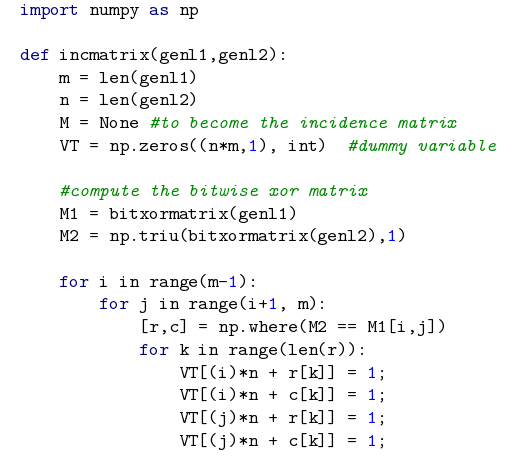The syntax to set a colouring style is easy, the command \usemintedstyle{borland} uses the colour theme borland to format the source code. You can find more colour schemes in the reference guide.   Open an example of the minted package in Overleaf  Captions, labels and the list of listings Code listings formatted with minted can be included in a float element, just like figures and tables. Captions and labels can be assigned to code listings, and then later be referenced and included in a "List of listings". \begin{listing}[ht] \inputminted{octave}{BitXorMatrix.m} \caption{Example from external file} \label{listing:3} \end{listing}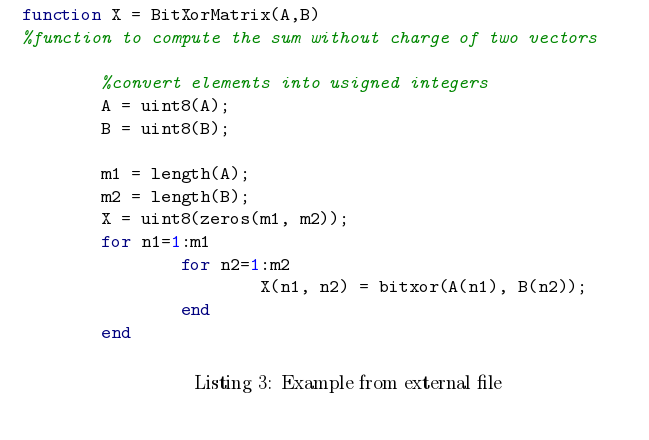To print the list with all listing elements use \listoflistings \renewcommand\listoflistingscaption{List of source codes} \listoflistings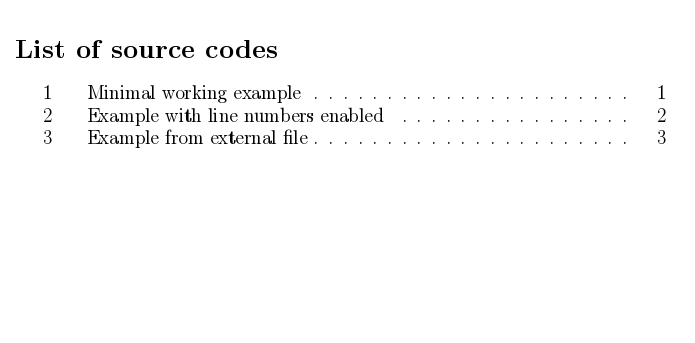In the example above, the default title "List of listings" is changed to "List of source codes" by \renewcommand\listoflistingscaption{List of source codes}   Open an example of the minted package in Overleaf  Reference guide Colour styles for minted name output name output manni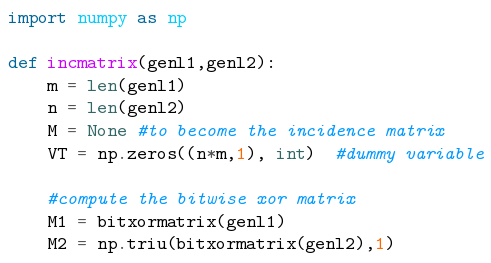fruity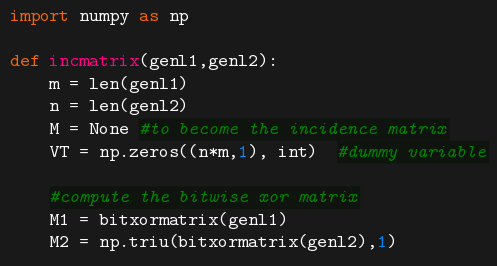rrt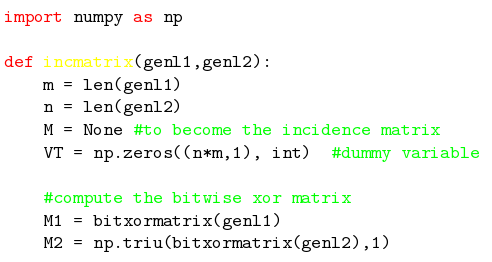autumn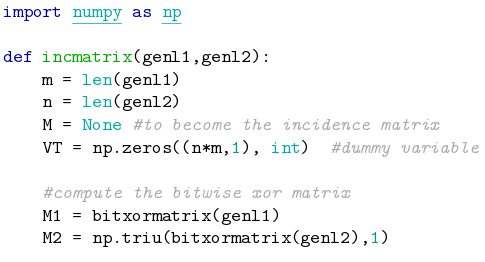perldoc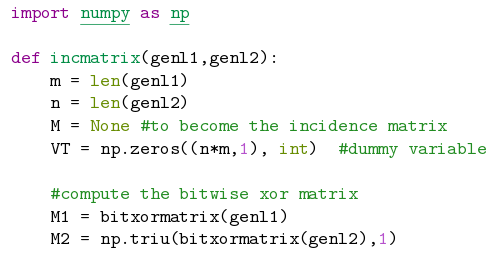bw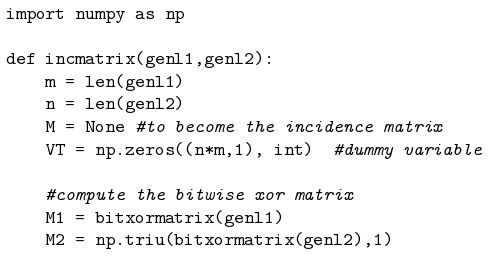borland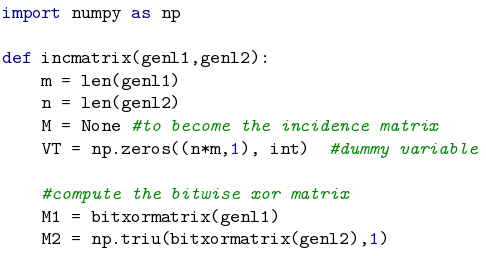emacs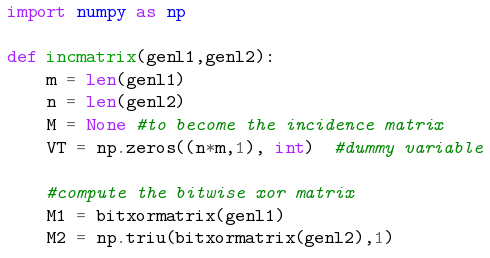colorful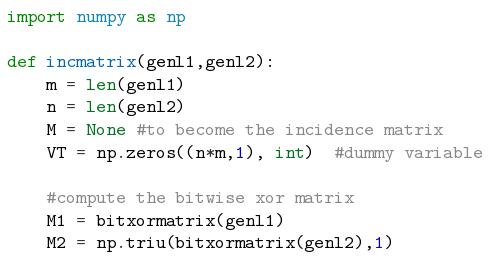vim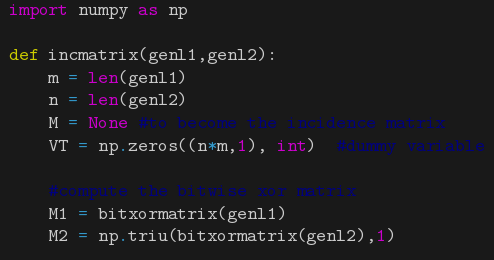murphy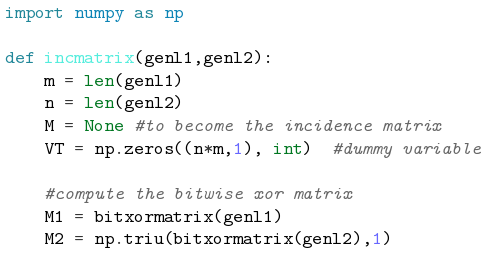pastie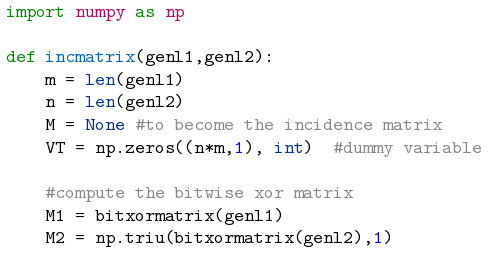vs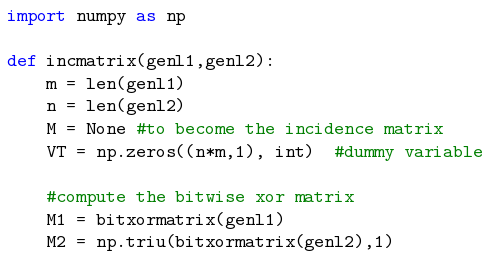friendly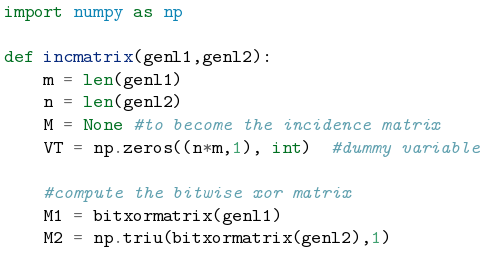trac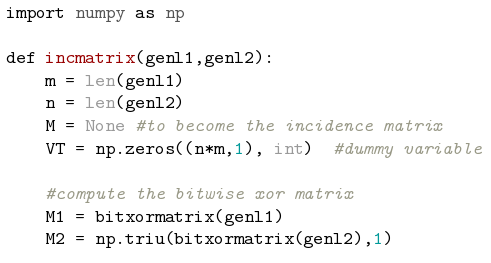native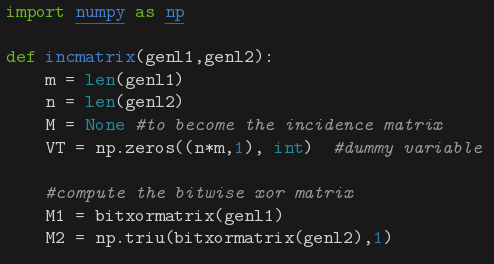tango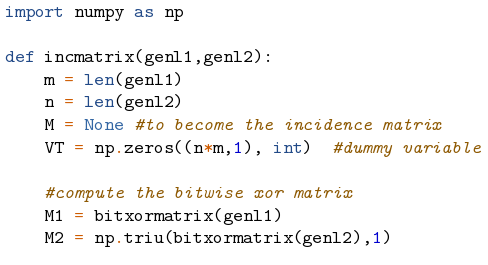monokai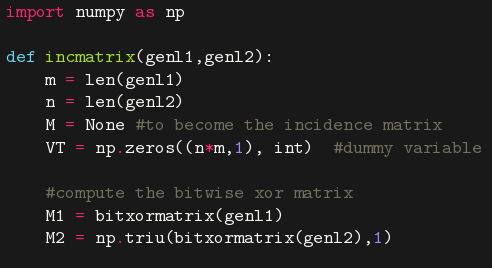Some colour schemes need a dark background to be readable. Main supported programming languages and configuration files cucumber abap ada ahk antlr apacheconf applescript as aspectj autoit asy awk basemake bash bat bbcode befunge bmax boo brainfuck bro bugs c ceylon cfm cfs cheetah clj cmake cobol cl console control coq cpp croc csharp css cuda cyx d dg diff django dpatch duel dylan ec erb evoque fan fancy fortran gas genshi glsl gnuplot go gosu groovy gst haml haskell hxml html http hx idl irc ini java jade js json jsp kconfig koka lasso livescrit llvm logos lua mako mason matlab minid monkey moon mxml myghty mysql nasm newlisp newspeak numpy ocaml octave ooc perl php plpgsql postgresql postscript pot prolog psql puppet python qml ragel raw ruby rhtml sass scheme smalltalk sql ssp tcl tea tex text vala vgl vim xml xquery yaml  Further reading For more information see: Lengths in LaTeX Code listing Counters Using colours in LaTeX Font sizes, families, and styles Font typefaces Management in a large project Multi-file LaTeX projects Table of contents Single sided and double sided documents The minted package documentation